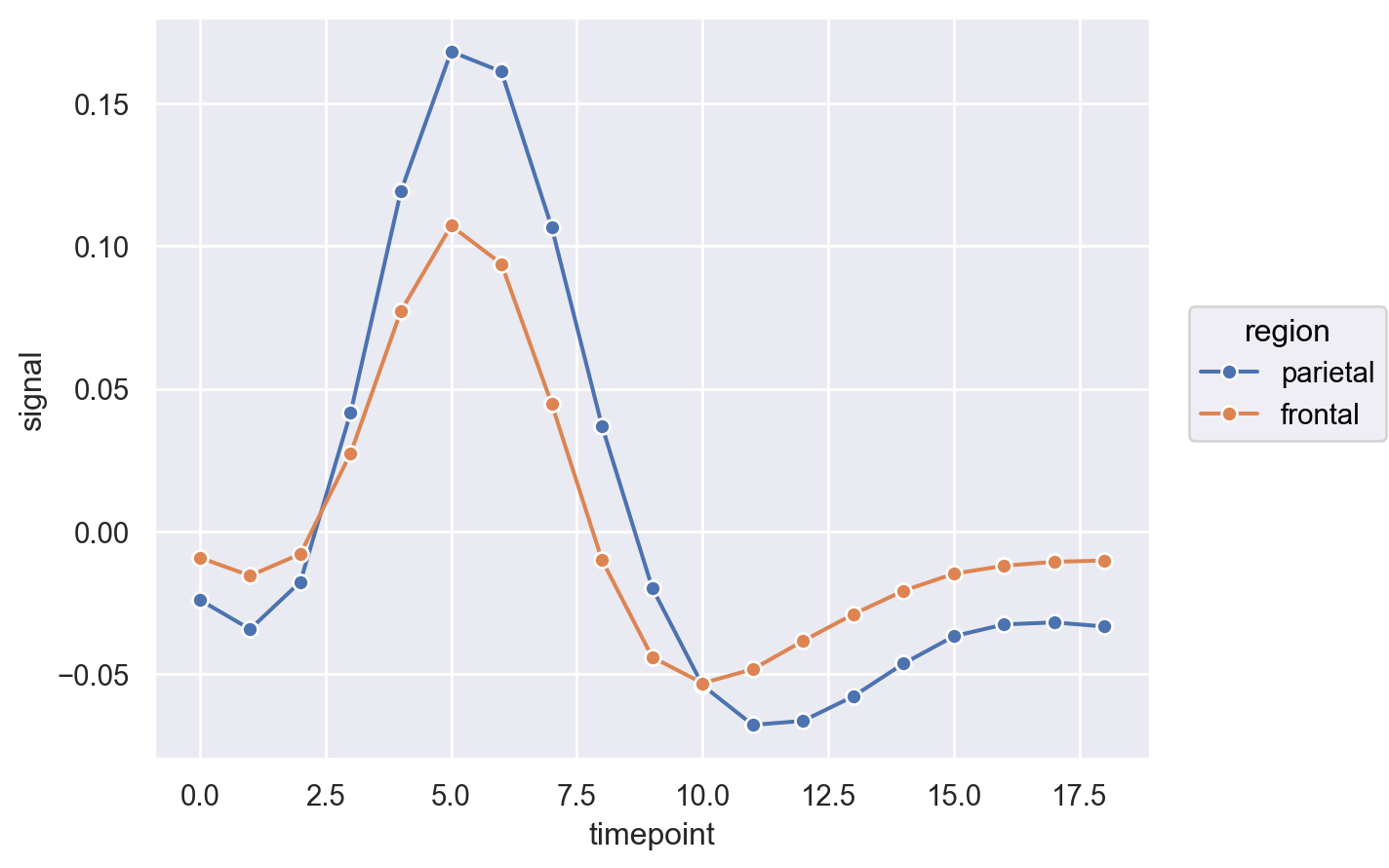# seaborn.objects.Line#

class seaborn.objects.Line(artist_kws=<factory>, color=<'C0'>, alpha=<1>, linewidth=<rc:lines.linewidth>, linestyle=<rc:lines.linestyle>, marker=<rc:lines.marker>, pointsize=<rc:lines.markersize>, fillcolor=<depend:color>, edgecolor=<depend:color>, edgewidth=<rc:lines.markeredgewidth>)#

A mark connecting data points with sorting along the orientation axis.

This mark defines the following properties:

`Path`

A mark connecting data points in the order they appear.

`Lines`

A faster but less-flexible mark for drawing many lines.

Examples

The mark draws a connecting line between sorted observations:

```so.Plot(dowjones, "Date", "Price").add(so.Line())
```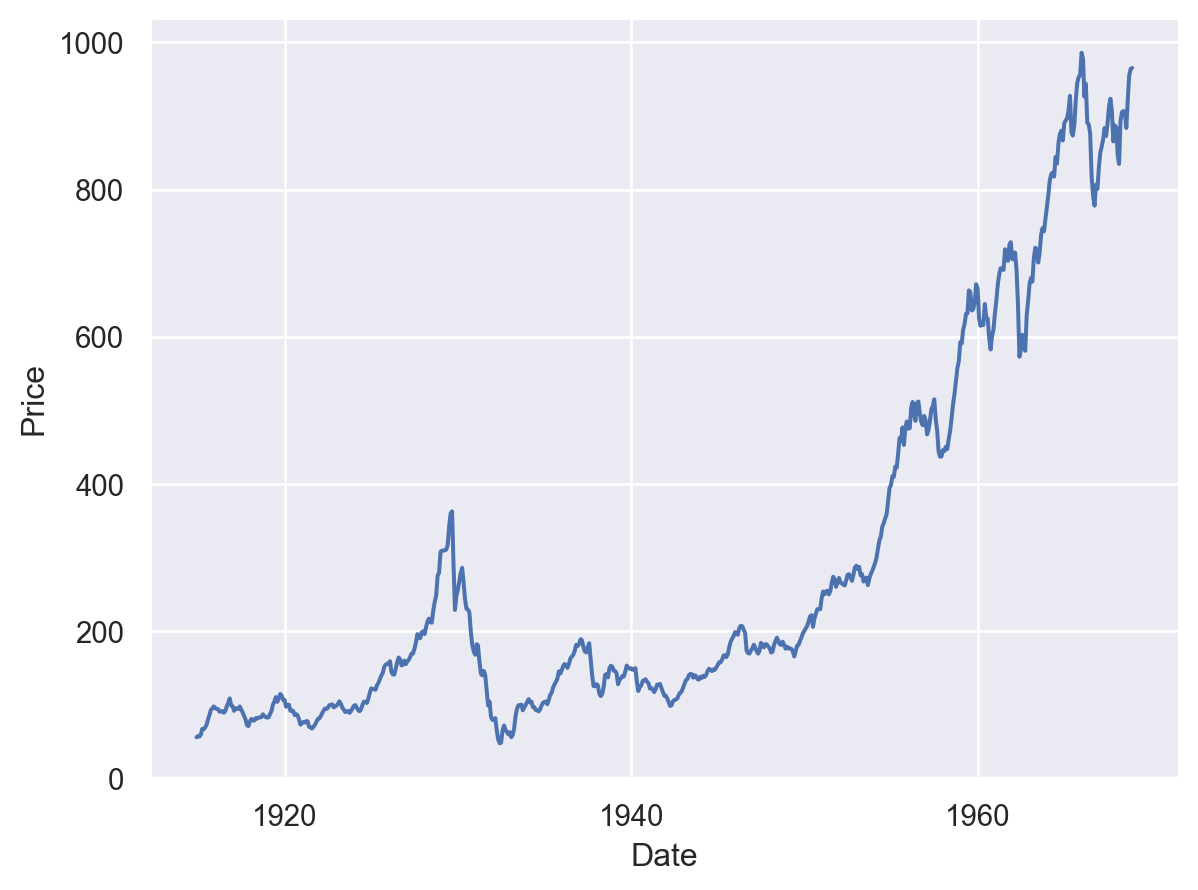Change the orientation to connect observations along the opposite axis (`orient="y"` is redundant here; the plot would detect that the date variable has a lower orientation priority than the price variable):

```so.Plot(dowjones, x="Price", y="Date").add(so.Line(), orient="y")
```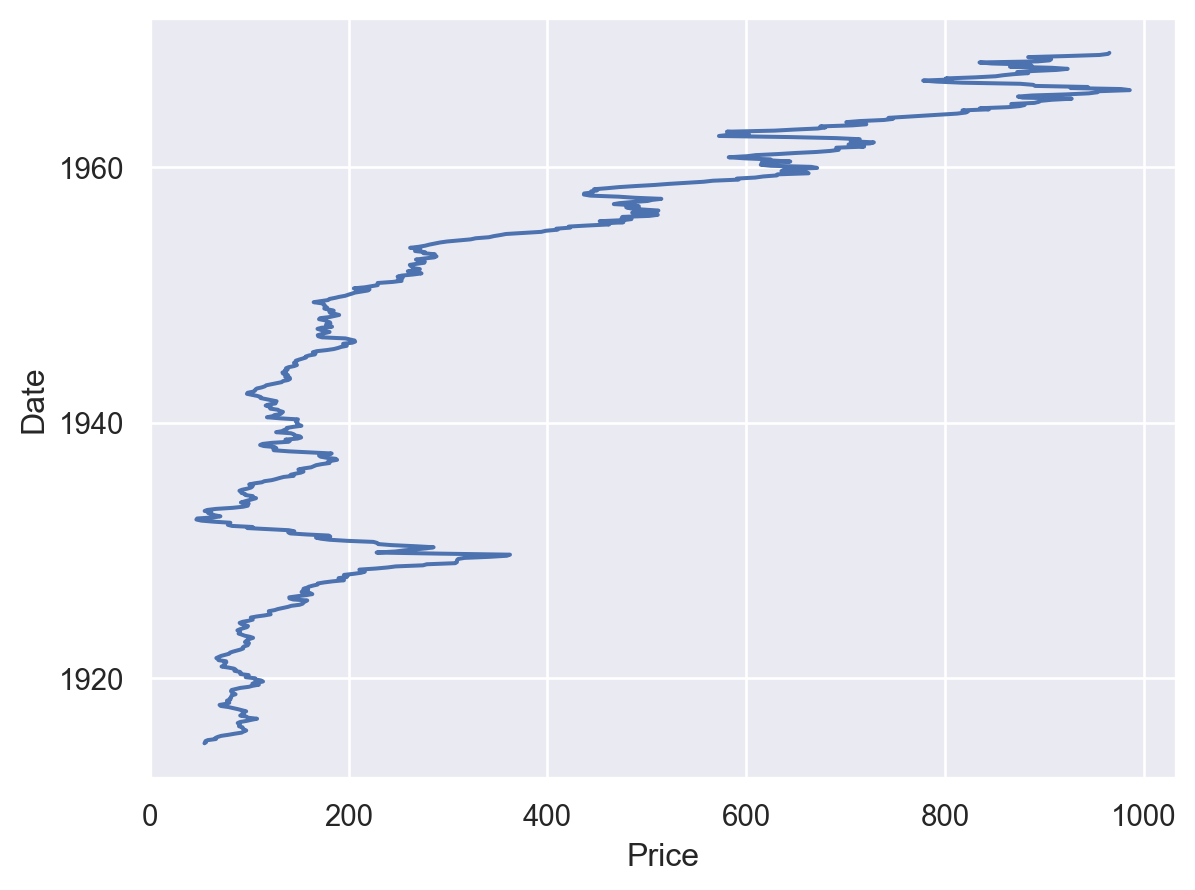To replicate the same line multiple times, assign a `group` variable (but consider using `Lines` here instead):

```(
fmri
.query("region == 'parietal' and event == 'stim'")
.pipe(so.Plot, "timepoint", "signal")
)
```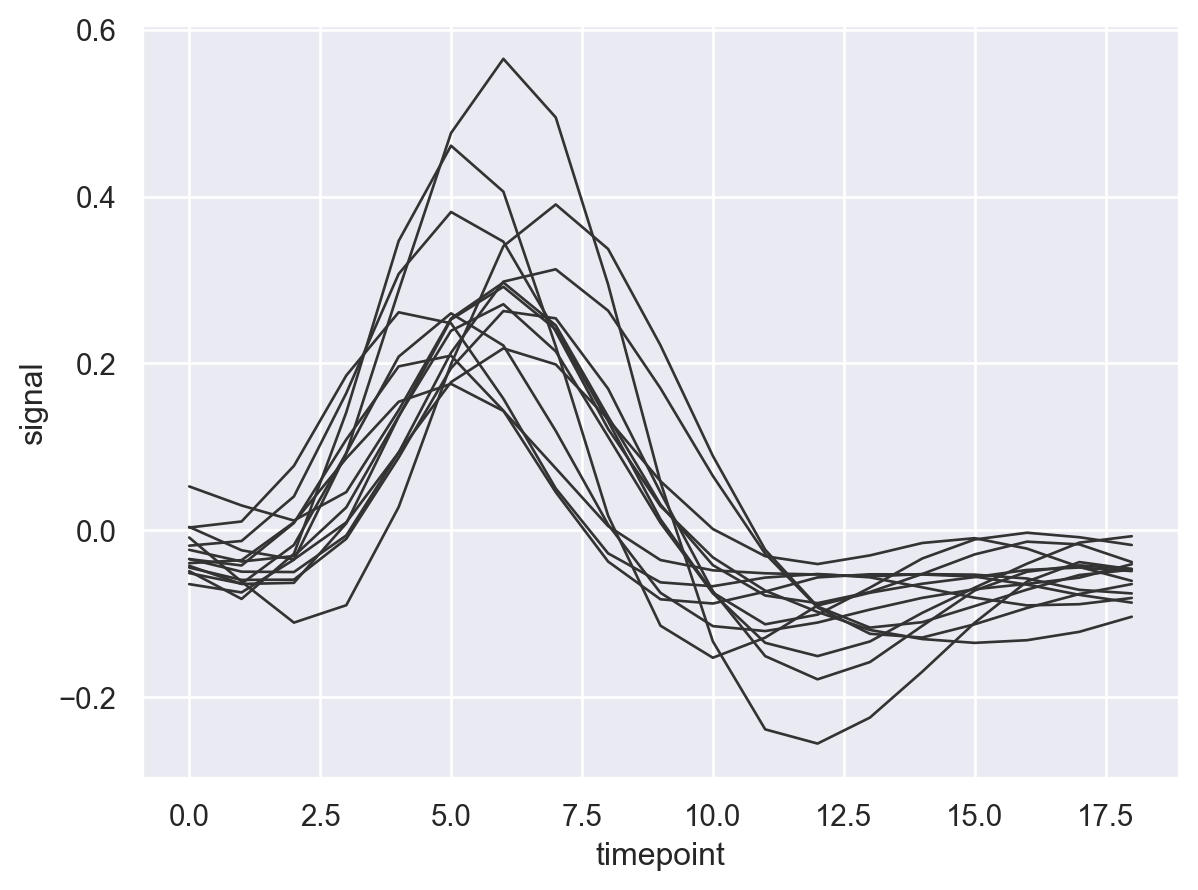When mapping variables to properties like `color` or `linestyle`, stat transforms are computed within each grouping:

```p = so.Plot(fmri, "timepoint", "signal", color="region", linestyle="event")
```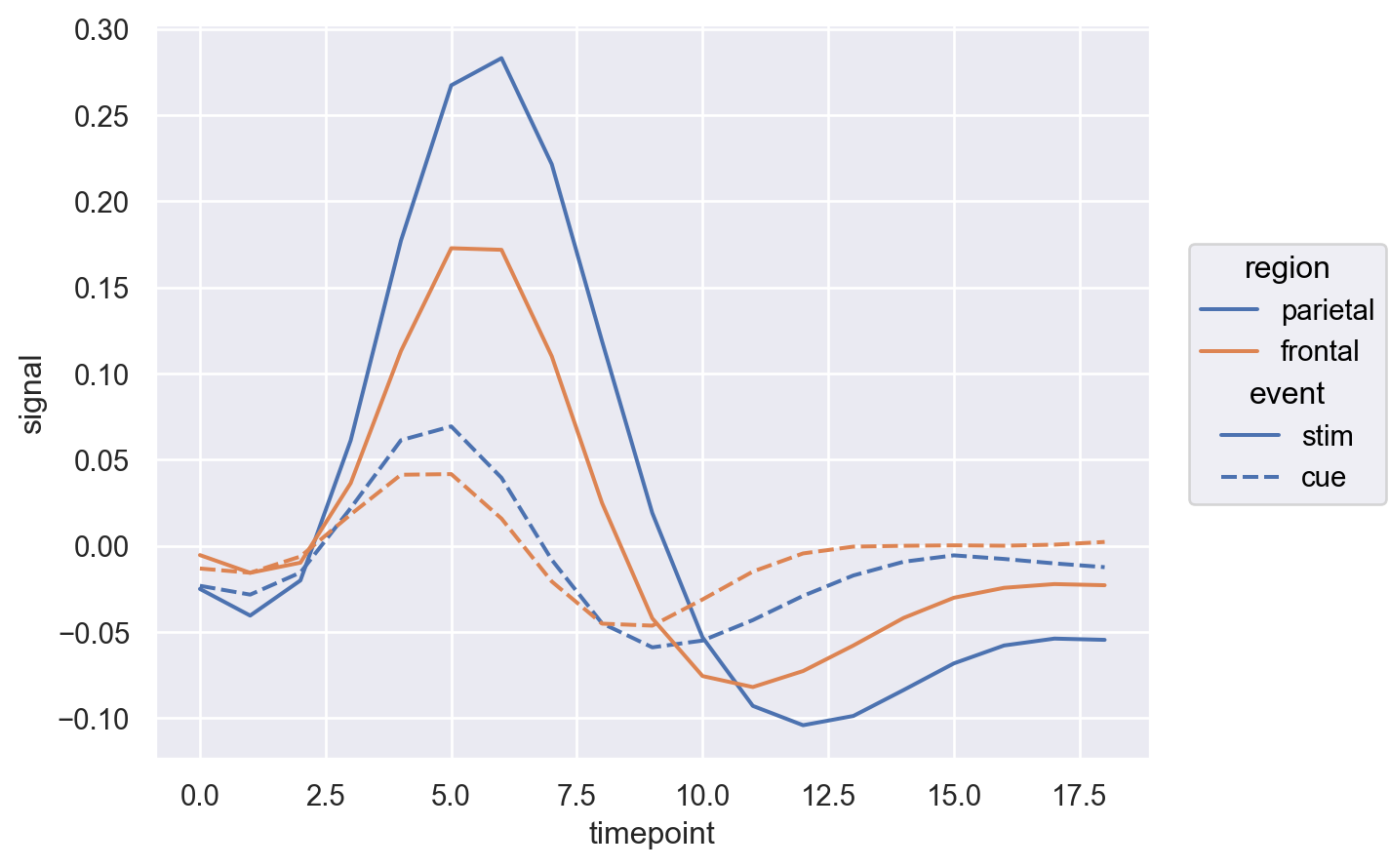Combine with `Band` to show an error bar:

```(
p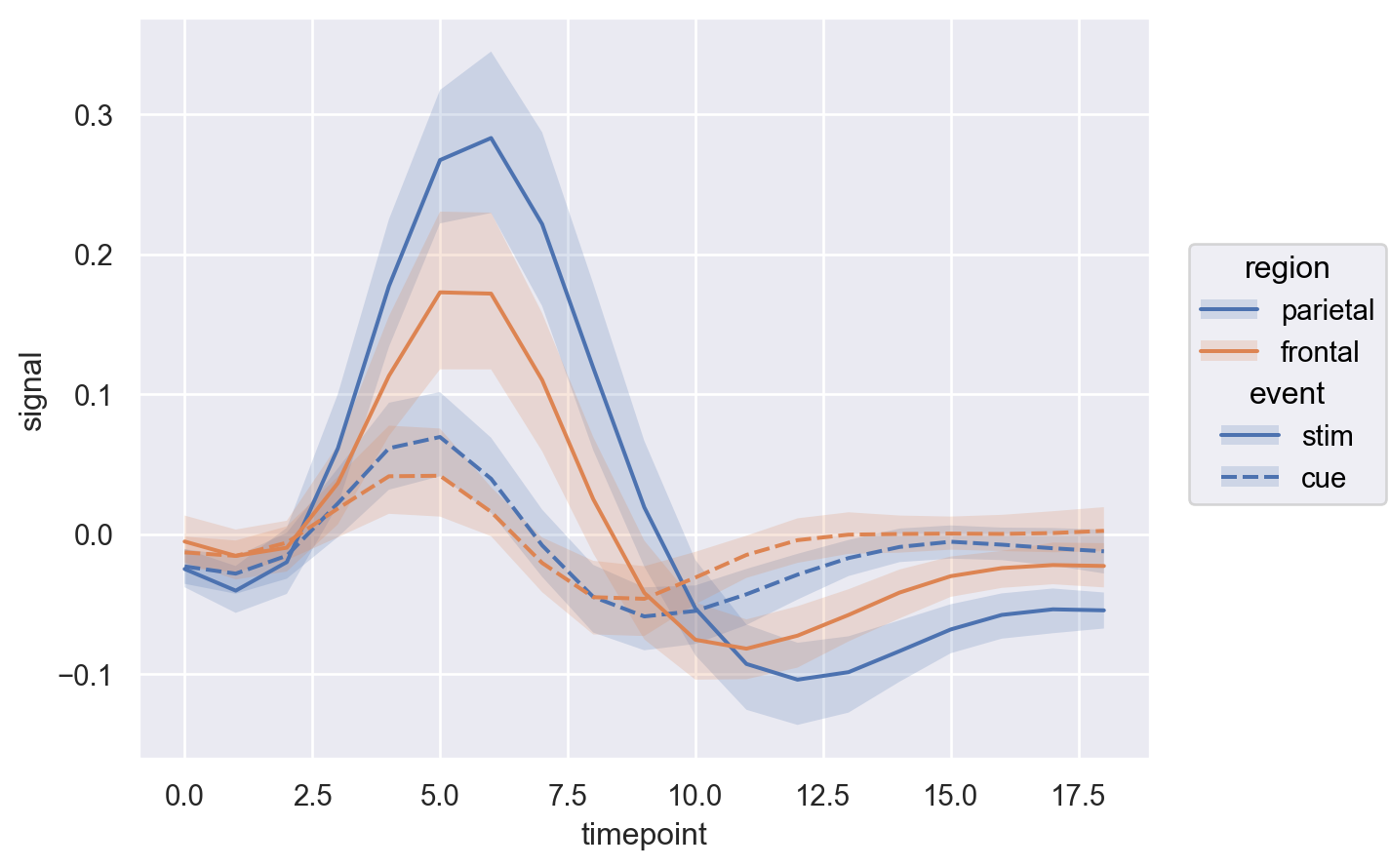```p.add(so.Line(marker="o", edgecolor="w"), so.Agg(), linestyle=None)